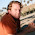## Monday, March 14, 2016

### Woes of the number 1.0

So, here I am, programming away on my path tracer. All is well in the world. When all of a sudden, I notice that after ~3 seconds of rendering (it's a progressive path tracer), black pixels start popping up on the screen. (One on the green sphere, a whole chain to the left of the bottom blue sphere, etc.)

Uhhhh, WHAT?!? WHY?!?!?

This is really odd, since a progressive path tracer is pretty much a running average, so how the hell can I get a perfectly black pixel pop up out of nowhere? So, I slapped a conditional breakpoint on the framebuffer color splat, using the exact pixel coordinates of the black pixels, and sure enough, NaN color.

Ok, that's all fine and dandy, but how am I getting a NaN color from the integrator? "Okay, look for all the things that can make a NaN......... AHA!!"

// Get the new ray direction
// Choose the direction based on the material
float pdf;
float3a normal = normalize(ray.Ng);
float3a wi = material->Sample(ray.dir, normal, sampler, &pdf);

// Accumulate the diffuse/specular weight
weights = weights * material->Eval(wi, normalize(ray.dir), normal) / pdf;

That divide by pdf looks suspicious. If pdf is zero, then weights would be NaN. Ok, so let's dive into Sample()

/**
* Creates a random direction in the hemisphere defined by the normal, weighted by a cosine lobe
*
* Based on http://www.rorydriscoll.com/2009/01/07/better-sampling/
*
* @param wi         The direction of the incoming light
* @param normal     The normal that defines the hemisphere
*
* @param sampler    The sampler to use for internal random number generation
* @return           A cosine weighted random direction in the hemisphere
*/
float3a Sample(float3a wi, float3a normal, UniformSampler *sampler, float *pdf) override {
// Create random coordinates in the local coordinate system
float rand = sampler->NextFloat();
float r = std::sqrtf(rand);
float theta = sampler->NextFloat() * 6.28318530718f /* 2 PI */;

float x = r * std::cosf(theta);
float y = r * std::sinf(theta);
float z = std::sqrtf(1.0f - x * x - y * y);

// Find an axis that is not parallel to normal
float3 majorAxis;
if (abs(normal.x) < 0.57735026919f /* 1 / sqrt(3) */) {
majorAxis = float3(1, 0, 0);
} else if (abs(normal.y) < 0.57735026919f /* 1 / sqrt(3) */) {
majorAxis = float3(0, 1, 0);
} else {
majorAxis = float3(0, 0, 1);
}

// Use majorAxis to create a coordinate system relative to world space
float3 u = normalize(cross(normal, majorAxis));
float3 v = cross(normal, u);
float3 w = normal;

// Transform from local coordinates to world coordinates
float3 direction =  normalize(u * x +
v * y +
w * z);

*pdf = dot(direction, normal) * M_1_PI;
return direction;
}

It creates a cosine-weighted random direction in the hemisphere. Hmmm, the only way for pdf to be zero is if dot(direction, normal) is zero. Aka, the new direction is completely perpendicular to the normal.

Ok, so if direction is perpendicular to normal, then z == 0.0 (since x, y, z are locale coordinates relative to the normal, where the normal == z). How can we get z == 0.0?

Like this:
float rand = sampler->NextFloat();
NextFloat() will return a float in the range [0.0, 1.0]. So, let's suppose it returns 1.0
float r = std::sqrtf(rand);

r = 1.0, since sqrt(1.0) == 1.0

float theta = sampler->NextFloat() * 6.28318530718f /* 2 PI */;

float x = r * std::cosf(theta);
float y = r * std::sinf(theta);
float z = std::sqrtf(1.0f - x * x - y * y);
Since r = 1.0, x * x + y * y will always equal 1.0, so z == 0.0

This is really annoying! It makes perfect sense for 1.0 to be a valid random number, but it completely destroys this particular algorithm....

So, we can either check for 1.0 and reject it, or redefine our random number generator to only generate on [0.0, 1.0). Sample() is going to get called ALOT, so adding a branch is no fun. Granted, branch prediction is going to give us a big help, but still seems kind of gross.

So I went for the latter approach, and fixed the random number generator to [0.0, 1.0). This seems to be the standard for other mathematical things, so perhaps there are other algorithms that don't play well with 1.0?

So in the end, random black pixels on the screen were caused by:
1. Random number generator procs a 1.0
2. The cosine-weighted sampler can't handle 1.0, causing the pdf to be 0.0
3. The pdf is later divided through the sample (as per Monte Carlo integration)
4. Which causes a NaN
5. And since anything added to NaN is NaN, the pixel is now permanently NaN.
6. When the frame buffer is passed to OpenGL to render, it interprets NaN as (0.0, 0.0, 0.0, 1.0)

-RichieSams

#### 1 comment:

1.This comment has been removed by the author.